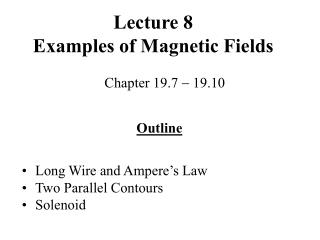DownloadDownload PresentationLecture 8 Examples of Magnetic Fields

Lecture 8 Examples of Magnetic Fields

Télécharger la présentationLecture 8 Examples of Magnetic Fields

- - - - - - - - - - - - - - - - - - - - - - - - - - - E N D - - - - - - - - - - - - - - - - - - - - - - - - - - -
Presentation Transcript

1. Lecture 8Examples of Magnetic Fields Chapter 19.7  19.10 Outline • Long Wire and Ampere’s Law • Two Parallel Contours • Solenoid

2. Magnetic Field of a Wire Charges flowing in a single wire produce magnetic field, whose field lines circle the wire in the directionaccording to the right hand rule. The magnetic field strength (B) is the same at all points of the circular path around the wire. B at distance r from a wire carrying current I is: 0 is the permeability of free space 0  4  107 T m / A 0I B =  2  r

3. Ampere’s Law Andre-Marie Ampere proposed how to calculate the magnetic field in an arbitrarily shaped wire. Ampere’s circuital law states that over any closed path around the wire (B‖l) = 0I This law gives an identical result to that for a long, straight current. Ampere’s circuital law is only valid for the currents and fields constant in time.

4. Magnetic Field Between Two Parallel Conductors Two current-carrying conductors exert magnetic forces on each other. Consider 2 long, straight wires of length l carrying currents I1 and I2 and separated by a distance d. 0I2 B2 =  2d 0I2 0I1 I2 F1=B2 I1 l =  I1 l =  l 2d 2d

5. Example Two wires positioned one above another parallel to the Earth’s surface have a weight per unit length of 104 N/m each. If they are separated by 1 cm and not affected by Earth’s magnetic field, what must be the current (same in both wires) to keep the upper wire in the air? mg 0I2 = l 2d 0I1 I2 F2 = l 2d F1 = mg I =  (2d mg/l0) =  (107 dmg/2) = 2.2 A

6. Two Parallel Conductors The magnetic force exerted by one wire onto the other is directed toward the other wire. F1 0I1 I2  =  l 2d Thus, the wires carrying currents in the same direction attract each other. This result is derived using the right-hand rule No 1 and in compliance with Newton’s third law. Definition of the unit of current, ampere. 1 A is the current in 2 wires 1 m apart attracting each other with the force per unit length of 2 107 N/m. All magnetic fields originate from moving electric charges.

7. Magnetic Field of a Solenoid Solenoid is a coil of several closely spaced loops. Consider a solenoid of length l and total number of turns N carrying a current I. The magnetic field inside the solenoid will be: B = 0 n I n = N/l

8. Current in a Solenoid Problem: A superconducting solenoid is designed to generate a magnetic field of 5 T. If the solenoid winding has 1000 turns/m, what is the required current? B I =  0 n n = 1000 B = 0 n I I = 5/(4107 103) = 3978 A

9. Ampere’s Law for a Solenoid The magnetic field B inside a solenoid is uniform and parallel to its axis. B outside a solenoid is virtually zero.  (B‖l) = BL = 0 N I Picture of a solenoid field N B = 0  I = 0 n I L

10. Ampere’s law is a relation between the current in an arbitrarily shaped wire and the magnetic field produced by the wire. Summary • Magnetic field of a closed loop increases the magnetic field set up by a piece of wire.